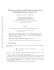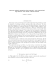# Full text

## Transcription

Full text
```dbsmact, Let (X,,) be a squence of independent real vatued random variabl~s.A suitable convergk:ncecondition far affine normalimned
maxima of (XJ k @given in the seBLjstable setup, i.e. fur inmasing
samplirdg squeaws. (kJ such that k,, 'Jk, 4 c > 1, which enabl~sus to
abtaia a hemigoup stwcture in the h i t . We show that such h m gaups are domly related to max-semi~Ifdocampasab1elaws and that
the nomjng sequences of the canvorgence condition ccan be choseri
such that the &miting behaviour for arbitrary sampling sequenms can
be fully malysed, f i i s in turn enables us to obtain r a n d o m i d l h i &
as follows. Suppse that (T,) is a sequence of positive integer valued
random variables such that %/kt&or TJs canverges i a probability to
some positive random vwiable D, where we do not amumt: (JC,J and
(T,] to be indepadent. Then we& limit theorems srf r a n d o d d cx\$remesswhere the sampling SequeacP: (Ic,) is ~eplacedby tiuldom rample sizm (9,
are presented. The prod follows cort-espondlnh:resuits on
the rxntral limit thcnrem, mataining 'che wmcarian of an An%ornk
condition.
Key wards nind phrases: E x t ~ m ~values,
e
max-semistable disbihukions, hcmigoup, rna~-sc2lnisclfdecampombiIity,random ~arnpiaiw,
,
Let X X , ... be independent, not aecegsarily identially distributed real
valued random variables and M, = mitx(XL,. .., X,), kssurne that for some
h~rmsingsequence (kn)of natural numbers and n c l d n g mnstants a, > O and
b, E R the dfine normalized maxima a, (MR,,-\$,J convcsgt: in cliistdbution ta
some aendcgenerate limit. AS argued in [9j3 the mast general hmewsrk, 111
w11ich sadsfgring reswlls far weak convergeam can be obtahed is: to assume
k,+,/k,, 4 c 1 for the growth of the sampling sequence. If additionally the
sequence (X,)of rdndam variables is ider3ti:icaIIydistributed, the cases c = 1and
e -s- I refer to max-stable and max-sedstabla H ~ t s r~specZively;
,
see e.g, &4]
and CS]. For non-identically distributed random variables and k, = n tbe limit.
is max-selliEecolmposab1e; see J31,
Momover, is3 [I161 a fuijlactional limit: law
for all t 0,where G,is a nondegeaesate probability distribution function @df)
and convergence holds for all points of continGQ x sf G,, is wxumed to obtain
certain seIfsimilar extremal proasses in the W t . I-f~sler bas abtdnecl
similar results for multivzlPiate extremes by assuming additionally an i&nite~imality condition. to avoid eases where the maxima are dominated only by some
of the random variables. Up ta now, neither the max-semistable Fase nar the
rnax=smise~fdeeompo\$'dbleone for non-identiedy distributed random wadab l e ~has been considered in the literature. En the next section we preslent
s mitable convergence candihomt,which natupdy extends (1.1) to seJnishMe situatiam, i.e. to situations where Emits of nomalizd m a x b .can only be obt&Ex?l
along s a q l i n g sequences [k,,) with the growth condition
\$/&-+ c 1, We
show that the structure of the Iirnits fits into a hemigroup setting with a close
c o a n w ~ o nto max-seoliselfdceomposabilify. We r e s l ~ c our
t mnsiderations to
one-dimensional extremes. Moreover, the norming sequenas fulfil an e m M ding property that enables us to dek
the limitJng beha.IYiour for arbitrary
sasnpbg sequences as well as for random sarnple sizes, The latter is understood as hllows,
Let (TI)he a sequencx of positive integer valued random variables defmcd
on the same probability space as (X,)and consider Tche ra~~domized
maxima
MT,= max (XI, ...,XTR))
where we do not assme (X,J and (Ti)
to tae iadevndent. Under the condition K / n + D i s probability, where E) > O is an arbilraw
positive random variable with diskibutiow Q, randrjmixed limit theorems were
obtained for domains of attraction of mmx-shble distributions; see , El21
or [4J Br-i particulx, if (X,)is an i.i.d. sequerlce and
for dl points of ccrndjnuity ?G of a noS1Clegellcrate piif G, then G is max-sbbk arid
under the almve-menfialled aaumptions on the ra~~dom
sample S~FESfQwe have
.rS
P (MT,
4 c ~ x, -t- b,)
Gyx)d~ (tIs
-t
0
where here and ia what hllows, according to weak tapalog_y,canvergeace of
pdfs is meant p o h ~ s efor every pint of e o n t i n ~ t yof the limit. For more
genera3 msrrlts on extremd processes of tritanalar m a y s of rowwise i.i.d. variables we refer to 11151. h eorapalrisom of our metbods and results ta those of [rq
Is given in Sectien 4.
We will gneralize (1.21 ta noa-identieally distributed random variablm
and semklable situations. These randomnimd limit theorems are pxsented in
Scc~on3 where, unlike the proof of (1.2) in  or , we follow the ideas of
carregpanding resulls on randornkd f i ~ t for
s nsrmdized sums of indepadent random variables. A randomhed central limit theorem has been proved in
thrm mnsaulive steps: first for random sample sizes z8 LrtiD_I with discrete
random vafiable D > 0,and next far TR,h-* D in probability, a g ~ i nfor discree
D > 0, both by Rdtlyi [IO]; the last step far arbitrary D O was shown independe;ntlg in [?] and El?], Meretts in the finst ste;p a mixing property sufiices,
the last two steps require an Anscornbe condition (see [U) to be fuEfled. "&'his
condition is of independent hterest and shdl motivate our method of prod
Note &at. if ( P;)i is a nondegeaerate max-stable extreanal process independent of D with correspoadhg pdfs .(G8]c,o,then the limit in (1.21 is the pdf
of Y''. This asymptotic independence is a consequence of the mking property
PIE: win derive far the given more gerreral situation in Proposition 3.1.
I;,.=.
As before, let fXJ be a swuenee of independent, not necessasily identically
distributed real valued randam variaMes and for all Q G s < t aud n f N de5ne
Moreover, let .Fz3be the gdf of he!:*. Suppose that for some Lnerelas-ingsequence
(k,) of naturd numbers with kk,+,/k, -+ C. > X and normiag consants a, > O
and b , ~ &we have
for aIl stquea-
O g s,
< 1, with s, -+ s,
t,
-.t, s < t, and some nnxadegenerate
N'G*J*
P~opos~a.lo~
2.1. The Gwiu GBB,in (2.1) cantinuaumrly depend OR. ~hr?pa~mxeters0 G s € t with respecl to weak topulosjry,
Proof. Let O G ,s < r, with s , + s , 8 , - t , s .+r k and E > 0 be arbicmry,
Let x E lf4 be a point of cuntbuity of GB;;,,alld choose 6 > O such that x d i s &o
a point sf ctrntinuity and G,,(x S] - C;,,, (x):.r c/B. Ckoase 0 G i5m4 6 S U G ~
that a:a- 8, is a point of ei)x~ikinuityof Gsmtlm
and G
,,,,
(4<: \$3 Since
+
chouw a m e N s ~ that
~ hfbr dl a 3 ur, we have
+
Without loss of generality we can choose rn I---+ n, to be strielliy increasing, Thcr.~
we have
lim sup .F\$;irn
,lt(
(x +5,)
nt -"03
+b,,)
c-G C;,,,(x -k 53
and
such that for all sufihently large m E N we get
Hence kbr suficiently large r n N~ we obtain
which proves the continuity of C,, on the set sf parameters. s
In view sf Propsition 2 4 quivalenly to (2.1) one may say that the dfine.
(M?: -B,,) converge: in distribution to some nerndenormalized maxima
@aerate limit unifarlnly on compact subseb of {O d s < t). The followirug
remark-ks relate this assumption, on uniformly wnrp%et convergence to weU-known convergence conditions in spe&al situtions,
Re rn ar k 2.2. To compare (2.1) with the functional h i t Iaw (1.1) let us
exaptionally assum k,,-- n in contrast ta the growth condition on the sampling sequence. According to Weissmr4.n [f Tj the lnoming constants in (1.1) can
hie chosen such that for some a E R and all k B we have
Especidly, tbr:sequence (an)can be chosen ta vary rregularfy, d thus ths: first
cotlvergence holds urrifonnly on mmpact subsets of ( t =- 0).amording to s\$mdard re;gulu variation technique; SEE e.g. C14-j. \$fie same t e e G q w s e m be
applied to see that also the latter wavergene halds unifomly on compact
subwiets of (t 0). 112 view of (1,1) we obtain far every sequence t, -t s > 0
-
-
As show11 in [I63 this is sufidcnt for (2.4) ta be fuffillcd with G5%,
= Go*JGo3,,
w h e GG,,
~ (x) is defined :dm be zero when Go-,(x) 0 even if Go, (x) 0. In [be
sequel we will derive similar results on the norming sequences as above, but we
need uraiformly mmpacl convergence to achieve the results in a more getleral
semistable setup,
R e m a r k 22. Suppose (X,,) is additionally identically distributed with
c a m o n pdf F* Then (2.1) is alreddy fulfilled if P; belongs to the d o m a h of
attrac~unof some nondegenemte max-semistable pdf G, i,e.
In particular, for dl sequences 0 6 ss, < t,, with s,, -4 s, t,
-+
t and s < t we haw
Bspecidzlly we obtain G,, = G'-"for
all B G s .= t. This shows that OUP results
m a be applied to the special case of ideatically &stributed random val-iables.
For a characterhation of the d m a i n of attraction we refer ta 171.
We lraw turn to the structure of the limits, which is determined by semistability and claselgr related ra max-semisalfdmomposab1e distributions,
P~o~osm
2.4.s ~Uptder the above-giuert assumptions WE haue
for all: O G s < r ..:t and x E \$
a,+ r
--z C'
A,
where a, P E R are determined by
and
b,+r---b,,
a,,
-+
8.
P r o of. Sine Fgt = P?;- \$2; for aII O .zg s < 1. 4 t and suficiently large
n & N , the first axsertiou, f d o w s by passing to lirnuits. Furghemnre, we kave
and on Ilrr: other hand
XHenee the rernaitiwg assertions ~OUBWby the convergence 0%'
types theorein; see
e.g.. Proposition 0.1 in 1211. B
~ ~ O P O S X T ~2.5.
qf .t2mpo.rition 2-4 the norrnilm
constants (aII)lalild (b,) can be chosen mrla tlmr eirker a -- 0 and & = log c or B: # 0
and Js" = 0.
% r nr~ f. Let us consider first the case -- 0, Suppose fi -= Q, Then we have
(xo+ fl c
446
P, Decker-Kern
Choose a point sf conSuppose j3 = 0, Then we have Gotl = C,, = Carl
such that 0 < Go,, (x,) < 1, H e n ~ efor & DIEN we obtain
tinuity x, of
1 CIS,(x0) = G,-I ,I(xO) Gc-m,,-rn* J (x~),v h i ~ himplies
*
-
=i
arrd mntradicts GO,l(xO) < 1. Hence must be positive.
Now define c, = a, @flogc r 0 md HS,g(x) .= Gs,(xP/jsgc), wXzjch is t l ~ e
nondegenerate limiting pdf of
for all sequences 0 g s, < t, with s,
naming constants fulfil
c,,1
-=-
C?)
an,, -+ c"
am
=
Z
and
-,s,
t, -s t, s < t. Moreover, the new
6,+1 -b,, Jogch + r - h t ,
-6,
-
B
can
-+
logc.
In the remaining case o?. p 0, define d, b, +sr, PJtl- cy aad a nondegenex dJ for itU sequenm8
rate Iimi ting pdf H,*, (x) = G,,,(x + 1 - cu]) of E;;",'" (azI
O d s, < lawith S, --+ S, t, -+ tl s <: t. The new n o d n g constants satisfy
+
This com\$etm the proof. a
D E P I N I T2.6.
T ~ In view OE Pr~gosili~ns
2-1, 2-4 and 2.5 we cdl the set sf
@F"s &? = (G,,,1 O d s < t ) a con~nuousnondcpnerate mux-semistable kemi~ ~ Q " in
O P
malogy
L ~
9s continuous convolution hmigroups far s u m a t i m schemes. Then thc ~~scquence
(X,) or the sequence of pdfs (F,j is said to belung to
the dotmin ~3f'ma~-.~mistabsC?
attraction of ,#. Moreover, by extendi~lgd ~ e
temliaalogy given in [9II far all t > O the elemcurs Goorof ,&' are m~ax-.~m~'zise&
dsea~~lposlable
in the full~wingsense:
Co~~versely,
wery -blondegenerate wax-semiselfdeeo~npossrhlepdf call lac
embedded into a \~-ontisuous
max-scunistnble h e m i p u p as we will show in the:
followiug
LEMMA2.9. Let G be a nondegmerate ~ x - s i e r n i s ~ ~ d ~ c n ~\$5
~ p ~i.e.
~~abie
*forsolne c 1, EE R and a lzoradegenevme pdf' H we have
tiphere B = 0 [fa #=0 sad fi = log G ifol = 0, BYI.ihoz~tIns8 6IJ"ge~ae~oElty
we assatme
T / ~ e nelen~i~v
N (x) = O ifG (x) = 0.
-
for nli! EN and some nor-mdegenerare pdf HIIwith HI = N avad H,(x) 0 if
G ( x ) = 0. The C D ~ Q C ~ O T(N,)[email protected]
S
E*,(x) -* Gfx) i j ct 3 O or \$ a < 0 and C htrs
no goitsit mass at 0, Moreouel; the cofactors ju&/ the C O C ~ C I L equdion
-
Obvious1y, G (0)= G (0)- H (0) such that eiti~esG (0) O ar H (0) = I,
Case 1 : &to)= 1.T11enNI(x)= 1,andhetacl:G(x)=C(~~;Y.)foraItll~30,
Thus 6 1x1 = I for 81241 x 2 O: holds true. Choose xo < 0 with O < G [xo)< I .
Then E (xo)= G (c'"x,) - Ift,(xu). Sii1cx: G (c""x,) 4 0, we musk have If,(x,) --+ m,
Case 2: G(0) = 0, Since I;fc""x)+ 1 for aU x > 0,we have N,(x) G ( x )
for aU x == 0. Mewaver, R,(x) .= 0 = G(x) for all x 4 0.
If rsl = 0, then h;(cn"x+np) = GCxf rtlogc) 4 1 for all XER such that
N,(x)a G (x) .
U ot < 0, then G @"'" x -+ n m = G (e-"lElx) -z G (6). As in the case a 3 0,
either 6 (0)= Zq OT. H (0) = I holds,
Case 1: E(0) =0, Cfaoase xo > O with O .= G(xo) < I. Then G(x,) =
E jcm:""
x,) H , (xu), aad since G (c"" x,) ---,G (0) = 4 we must have H,(xo) acl ,
Case 2 : H f O ) = I, Then G(x) 1 for all x 3 0 a above. %PtusIPn(xja G(xj
for ail x r R since G(ca"x) --+ G(O) = 1 by eontintlity of G Giltl x IJ.
Si;fgcxon the one hand
-j.
-
-
and OH the other hand
T I E E ~ ~2.8.
M Eerery ~nondrgenernte rnax=.~smise~decc~fnpo~~~b!c
pdf G2~12i~~'L"Iz
has PZOpoint ma38 at 0 \$'B: < 8, can be embecid~diintu a cu~slrf~auaus
max-sefizbrable hewaigmup 8-- {G,*,I O d s < 1 ) with G = Go,l.
F r o o f, In view sf the wfa'actors (H\$of Lemma 2.7 we define noncfcgenerate pdfls G,,for all O g s < a ;~tsfollows, For t > O write t = cnrr, w i ~ h11, ~2
and F'~E El, c), and define L, x = c-"@ x -n, ,8, Then we have
t , x = caL,,x+p
-
L,(eex+p),
and we further define:
where we set Ho zz l. It is easy ta verify that Go,l =. G.
I. v\re show first the hemigoup property: G,;G,, = 6;,,t.
If
O = 5 e v G t .f: c m ~ * we
l , have a, = n,, and hence
If IF
-= s cr. v < t < cnsf I , we have n, = n ,= a,, and hence
The Gases O < s < u i c b + ' 6 f and ~ < s < c " ~ ' ~ < o c = t < c " ~ "ca
' n 'be
treated similady. We prove the wrnainiag cast O < s < cna" ,( v .< lPw' G t
by applying the cocyclr; eqlmtioa of L m m a 2.7:
'
G,DWf%,* (4
- H (L x)""""/~r" H n,, - (me i- 1) CLu f-I r;Lw~ 1 " ' ~ ~
cs
x H [LC,
x)""""~~"I~
H rzr -(tall 5 I (-Zdt X ) (Let @'ogEp'
- H (1"c.p X)1""F(c/p8j , r , - ( n , + 1 ) C ~ u ~ ~ ~ C L c u x l P 4 , t - ~ itILtxx)-I"PCtcr~I'~~
n~~
+ 1, - cs, + 11 (kc,XI JJn, - (n. + I (Lt
(L
= ~r (EmX)~~'C'"""L"~
Q , + ~ I- ( ~ . - I - I I ( ~ - ' " ' " + ~ ' I , X + ( E ~ - ( ~ ~ + ~ I ) P )
x H,,- (,vV c 11 ILt H C L r x)'""~"
Ff (LC#
~)'"c~'"'
Hot,
H QLcs
H,,-,,(
+. 1, (L,
X) H
(LC,
x)"'~'" GS,,,
(x)
2. Since c ~ =
f . cwr" rt for all t r O and L,x -. L,, (8
x +B), we obtain the
max-semistability G,,, (c" x c @ = GK,,(x) for d O G s 4 r,
-
3. For contkuity of the hemigroup we first consider r, -t c and r, -+ c.
TE 0 s < t and rt -+r, we have
~ 'Goocat* i
Gas,(x).-+ G (A, X ) B (LC,x;) = G (45, X) G (LaX) N fLcztx ) ' ~ =r:
lf 0 < s < t r cop+h a d Y, -+C, we have fiS = n, an\$
G,, ( x )
-,H
-
(L, x)""sccctrst
[ LCS .&)108~k!%) Rt,,-5 1, -,,(
-
-- G8,PC t
PIU. other eases can be proved similarly. Finally let us comider continuity if
s -+ 0. Then we have la, i
.- oo, a d thus
-
* 1 ) (Let x)
if a - 0
co
(6.c21 x?lOfil
or L X > O and x > 8 ,
if c & < O or a , ~and
L,x--{O
X=O,
Y a > O and x<O.
[-KJ
We consider these cases for
G,,E(XI
= H fL, x ) " % ~H~nt-fn,+
~ ~ ~i f' (Lt~lH(Lct
x)iowt*
,,
From Lemma 2.7 we obtain H,,-( fL,x) -+ G(L,x).
Case 1: E,,x-+m. Then we: have
G,, (x)-+ G (S,x'j H ((E, x)""Ga,i (4Case 2: L,x+ -m. Then a , O and x < 0, in which case the proof of
Lemma 2.7 impties G (L,x) O since LL,
x < O. Thus
c;,, ( X I -.a = G (L,X) N CL,~ ) = G~ ~ ,EX,~). g ~
Case 3: L,,x-+O. If M ( 8 , we have HI01 = 1 as in the praof of k r n ma 2.7. Thus G, (44 GO,t(\$I as in lhe C B S ~1.
If ol r O and x 0,we have G (0) = 0 as in the proof:of Lemma 2.7. Thus
(0)as in the case 2. ira
G,,, -+0 = Go,,
We now prove some results on the tfsrmi~lgsequences (a,) a d (b,)in (2.1).
Namely, the* sewences can be chosen s u ~ hthat t b y can be embcddd into
sequences which have properties as in @2), The embedding sequences in turn
lead us to fully understmd the bmiting hhaviow; see aIsa C7J far the i,i.d. ease,
'Fke method of proof is similar to the one given fox domains of attraction of
semistable laws in the scheme af summatian; see [13f.
MM 2.9. Let Ehe normifig Goastanks (a?,)and {bJ be ch#ssn aecu~dirzg
l a Pmol~osition2.5, Tken rhere exist embeddl~gsequersces (c,) and (d3 with c, > 8,
-
;=:
Cb.,
= an,
dkls
bar @nd
~lnformlyon compact sabsef~;01{ A w8>. Especially, the segueace (a,) eari be
embedded info a regularly ltaryiprg sequence.
Proof. Write FZ== r,kw3 withp,~RFand k,,G n < k,,,r,
ejvely cen1pdc.t in [I, CJ Define
Thus(r,)issel;ia-
Obviously, we have c,, > 0, ek,, an and dkJ3= b,.
Let (;I,)
be a positive sequence wit12 A, -+ ; 1 ~ [ 1 ,c].
dl a s e I : Suppose p L 1,,,1 6 pn -2 along a subsequence In").Then wc have
I
;
:
in consadictiou to X 2 1.
C as;e 2 : S~lpposepl,,, = p,,- 1 along a subsequence (rr'l). Since (r,) is
rdativdy campa~t,every subsequence of (art) contajns a further subsequence
(sal'")with
r, -+T E [I C ] along (E'')), and thus along (n"') we have
In the case
e! =: 0
we further obtain
along the subsequence (n"), and in the case a
+ O we have dong
(?tl'")
%rice every sr_lbs~q:h~"cnee
of (n" anta fufrarrl~er subsequence (lz") with
these prugcrf;jes,wc get the assert& convergenGe results dong 4ke whole sabsequence.
C a s e 3:
= p , , J P ~ ~ , n=p,-i-l
j
QrpLn,,r--&+Z
dong a subsequence (53 can be tredted sidarly ta the =e 2 to obtain %he m e
cclrivcrgence results.
Case 4: Suppose p C J , , ~3 fil 3 along at subseque;mm ( ~ 7Then
% we have
+
50
I
+f c.
Maw let (2,J Itx: a positive sequeacr: with A,, -+ .
I u. Wnite R == yc-th
qr r [I, c) and p E N as welE ax; A,, =: y, c P with y, -+y. Then amordi~lgto t11e
above eases we have
and
Rndly, let (A,) be a positive sequence with 4, -+ [email protected], 1)-n u s 2-I > I and we
haw
and
This proves the theorem. m
T H ~ R B2.10.
M U ~ d e the
r co-rsditicms of Tffeorenz2 9 fafor all 0 G ss,< t, with
t , 4 t , s -+=t , the seqtreme ( 6 , (MF" -4,))is stuchastict.rl6j.l eompct", i . ~ .
the dissriblgtians are weakly relatively cornpat and all limit P ( P ~ ' P Iare
~ S nnodegene-
S, --, S,
rrmbe. Moreover, each pdf of the limit poirats beloags ro
\$ ct f O and
with r, -* r > 0, we herrlir
where pa,[;l)= O
.qgqesetxce
= logcZ,
In parFirrtfcrr., fi [r,) is a pagnsitiae
Proof, The last assertiom ~ ~ U O ' Vby
I I Theorem 2,9:
jpnrtr7
i k,,r;,j 4 ~ i a ~ r , ~ ~ + d ~ a , , r ~ 1 b
-%
and thus
G,,"*,
(r=" x S- ls,
(1'\$)9
To prove stocl~asticcompaetl~~s,
*rife again a = r.,k,, with P,EN and
k,, G rz < K,,,, . Thus (r,) is relatively comptrct in [I, 4, and far every limit.
point r E [I, c] we get, by the previous arguments, along a subsequence (PSI)
-
For r 1 and r ==- c the limit points coincide aaording to Propasitions 2.4
and 2.5. ~a
In this section we present randomimtion raults for the domain of attraclion of max-semistable hemigroups imludiag the proofs. Theorem 3.6, being
the most essential of these results, hscusses the limit of the ran
(a, l (M?",,
-b,)), where (TI)is a squence: of positive integer valued randam
varPrIaMea such that T,jk, -1. D in probability far some positive random variable D.
Note that xre do not assume (T,) and (X,)to be independent, neither reqwisc
informadion.on the dependen= strtructu~ebetween the two sequences, As s l a t d
in the Introduction, the proof follows conespoading results on the ~ e a r r dlimit
theorem. Therefore we give preparatory results relyling an eharacterie;rics of
mixing sequences of mndorn variables deveEoped by Rtrryi, as well as on Ammmbe's condition,
Prto~osr~ro~.
3.1. U~zderthe c~1ditio1asart\$ with the natation o j Theorem
A 0 and O < s r t the sequence (a, '(n/f'y:RAl-b,)) is ts mixing
2.10 for every
seqtrence of random ocariahks iua the sePtse of Rknyi, i.e. fur any evenr A with
pasitiue probability we have
P (C~
-b.1
~ : : l ~ d
.X
I A)
Ga8,a.rIxIe
-+
P 1-0
of. Let A, = (&fria,d
< cr, x s h,). Then by Lemma 6.2.1 rrf [44 it is
suficiel~tto prove for euerqr faxed m ~ P 4
Case t : 5. r 0. Hence L b k, AJ s j 3 L [kBbA ) Z] for sufficiently large
EN, and thus (3.1) holds true since then A, aad A,, are indcpnrdent evellfs.
Case 2: s = 0. Since
and hotb P(A,J and the latter prohitbdity om the kl'ght-hand side cullverge to
Gtjnl,(XI
for continuity points, we have
LEMMA3.2, Let
and ('2,)be sequences of positive integer valued random wriables with --, CC. a d Y,/Zn -+ L in prababil'ivfor.sof~tep ~ t s i t i ~rrzlae
d m oariable L. Thmz urude~the cofzditiomand with the tzuttrticln of 'Theorems 2.9
and 2.10 we have
CY,,
----+I?
and
4,-dzn-+ p, (E.1
Czm
i~ probability,
czn
Proof. Every subsequence (n3 contains a fwrher subseque;nce (a") such
that the event I"= { Y,J& -,L and Y, -+ ou alavg In'");)bas probability one. For
o f T Iet :t write %(a)= A, i(m)Z,(w) .= 2 , (~)Z,(~il)f
, and thus we obtain
An (a)--,L @) and 2, {mj -+m 3~~lang
(a'')). Hence Tor dl o s r it follows by
Theorem 2-9 that
eym'w'
4
L (w)"
PY~IU~
- d z n ~ ~ PO!(L(4)
and
+
czn(m)
+%m~~j
along (n"'). Since s bas probabdity one, the assertions hold true. aa
T H ~ 3.3.
R Urtder
~
the storzditi~asaPld with the notasion of Theorem 2.10
for all O G s r b the *followirzg Anscornbe canditi~nis fuEylilled:
PPOOEChoose al E(O, f ) with (2 + a d )s .= (1 -al)r s u ~ hthat far all
\$ a" we h;lvc jl-\$ii4a f S 8 ( s - a ~ r--.
< n i ' -< [email protected]*(x
and
n
thus we obtain
-= a 6 a, and Irri -nt
(32)
M
lim sup P { ma;x cI',
n-m
6 lim ssrzp P
Lt b b a
im~-n\Cm~
{e,
S
y
f
{MZi- M:')[ >E)
max (MiJ
-d)S3X
t
s
?)'-I
MtvfZ
3
e
+ c\$ ' M:']
454
P. Becker-Kern
Let us now consider the first expression of the last inequality an tbe
sight-ha~d side of (3.2). Define probability measures &A(I - a b , ~
I (
and
P ~ (i-aja,~4i
I
-up
respectively, Hence i t foUsws by Theorern 2.10 that the distributions af the
random vectors ( e l (Mi:- ")"a(1'"1"dJYc; (M,"ca)a,lz
-*lt -\$1) are stochastically compact and every weak limit point is cantahed i~
Causequet~tly,by the portmanteau theorem we obtain
6 Iinr sup f t (Ic,
-ubJ('
"ah
_-
I
(~
"")"(Ii ?
"Ifo>
+a13tlf7
is continuous oa
dPI)3 .+cn-
a-m
t'Or some it, E [I, c], siace AM
the podtive half h e . For a
-
-a)s,;r([email protected]
0 we have
a
Observe that For nU y E R
so that for every paint of ecl.nti!l~iir A\$x+h),(LO) of CJ,os,dor with
obtairi
Gnas,x,r(
As
-Ip,
20
(?Lo))
Let y , = i & f x ~ R 1 GGn,,sA,l(AQu~+p,(R,))r 0 ) ~ R u { - m ) .
Case I r y, > - a.Choose a, ~ (a,]0 such
~ that for all O < a < a, we have
Further choose y , E (yo, p, +44) S U G that
~
A\$ yl -I-p,:(AO)is a poiat of continuity
of * 6
,,,
E-o,, Then these exists a, ~ ( 0a,]
, such that
By (3.3) we obtain for all 0 < a ,< a3
C a s e 2 : yo = - m. Since (pAolI + n).s,ln(l
chcrose yl E R s ~ c hthat
-
i~ u n i f ~ d ytight,
Further choose y 2 ~ f yyk+8/2]
~ ,
such that Ah,y , +p,(Ao) Ss a paint of contimity of GxOhsxaf.Then there exists a3 E (0, all such that
Gn,,cl
as
-alx.norl i
(A; ya -I- pa (A,)) 3 1-618
far a11 0 < a 6 a,
in the case I. By (3.3) we s b t ~ afor all I1 < a 6 a,, as io the case 1,
456
P. Becker-Kern
Enafly, i~ both cases, for all 0 r. a g a, we obtain.
Andogonsly we can see that. for the latter expsesrsion on the right-hand side d'
(3.2) there exists ( ~ , E ( Q ,
swh tfiar for all O < a < a. we have
which tsgether with (3.2) and (3.4) gives; the asserted resuft, aa
The IoUowing lemma is proved in  and wil be helpful for further
results. The proof given in C33 is based on argunxents of Hilbert sgaee techniques.
LEMMA 3.4. Let &V,)
be a sequence ofindependent random variables and (k,),
[mJJbe two sampling sequences with JF, .g na,, k, --,co. If A, i s an menr depencffitsg
only on I\$,&, .,., YmVz,then for ar~ye~efatA we have
lim sup P (A, 1 A) = lim sups P (A,),
n-a
rz-m
where we sr;f P (A, I A) = P (A,) fi P (A)= 0.
The next bmma is crucial for the proof of r~ndomize-dlimit theorems.
Recall the three steps of proof for the randomized rsentral lirnit llleorem given
at the end af the Iz~ttztsoductian,Whereas in the first step 7;;= Lrar>_l xin nip;
properties are suffi~ient,in the last two steps approxi-matiom of T, by ~ R D J
with discrete DD,
and of arbitrary I) 3 0 by discrete rasdam variables are
applied, respectively. Anscorn be's condition defines the qlmalality of these approximations for randomized maxima as faHowsr
LEMMA3.5. Under tbze enaditicrvas and with rEle notation of/Ikit?~~ern
2.10 let
(U,)be a seguelace o f p o s i ~ v eintegcfi. eaalued rnrsdt,t;~tvariables wish U,/n -+ U in
probabablliry fbr so~nepositive ranl9om ncrrx'able U,
(a) i)f U is Bhtscrere, .for+all Q 6 s < r we harrc
(b) Far rn E M rt~fivne positive
dis.cl.ete mndom
nauiabtes Fn fry
&?lnarhe~,
for m, EN let Umt, he po,f.r'tiue integer ualued random uariabhs
gt'am by U,,,=. U,+ I n (rS,- U ) \$. Thrz-forevery G r 0 and O G s < r we ham
(3.51
h lim sup P ([cTiL-, j IM41m--4~
n-rm
ikf \$v,
111 3 E )
= 0,
Proof. (a) Let (uk)be a [countable) sequence with pk P ( E P = M&) > 0
and C , p k = I. For d > 0 cljoase N E N sUGh that x k Z N g
f iiSJZ. For any a a O
we have:
;--
Tkus with the events .En,&==
- b H U ~ JG a nu,
_/Iwe obtain
lim sup P (]~iT,~j
(Ma> MrAal)[> E)
I\$-* oJ
< 6/2+ ~1G lim
sup P (
N
fif a!
max
Im--lrrst*JISnLnuk
f
~ ~ ~ * - ~2 GI~ ~ w k ~ ~ l
Now choose ~ E ( O , 1) such that, by Theorem 3.3, for all k 6 N we have
6
limsupP{
a
l~~IkklI~\$,;'-JM~~,,I).I>~)~-.
n . 4 ~
Est-~vu~J1Gaim~*j
2N
Hence we obtain
lim sup P {reLt?l:uj(JM%a Il'rb
which prows (3.5) since E r 0 and 6 2 0 are arbitrary.
(h) We have 0 d Kz-iU < 2 -". Thm f~;t, -+ U in prabability. Further, for
any fixed EN we obtain
>, mU such that fer .dl pn 3 knit
Choose r n E~ N with P f U r mu) d 6/3 and
we have P ( U g pus-2-") ) \$613. By n e a r e m 3.3 fwther ehaose *I,3 rral such
that f ~ all
r w l > 1nz we have
-
Let pk (a) = 1usk 2-"1 iWLd de:frne events
Ek = {(Ck-f)2-Ffi < U < k-2-"1
-. {V,,
-
k~2-~'],
G I , , = ( ~ U , - P L ~ P ~ ~ I \$ P ckaE=(IUtr-~~Cn)l\$m-'pk(fi)]l
~=~-"~),
By Lemma 3.4 and f3,8), for all ICE N and s r O we have
lira sup P (Aks,1 Ek)G 15,13.
11-10
Fureher, far sufficiel~tlylarge n E N and k 3 m + 1 we obtain
and hence Gksnis contained in
we imply (3.6)
Gk,,. Using
the irelatioms
in the case s > O by (3,9), since for m 3 m,
WE:
have
In the case s = O we furlher I~aveto ~ ~ S G U Sthe
S application of Lemma 3.4
in (3.9). As in the proof of R~eurem3 3 , analogously to (3.2) we get
3im sup 1" (
I-bw
max
Il-p*tn)l<m
"8ttn)
< 2 linrrs-'rnsup P (e;,let
IC,{,~ (MP+'- Mi\$rlJ)l) 8 )
~~~~j"-'"".'l+*)
~ - E' "+ C F k- (L~ ) M ~ M O~. ( k~ c a-nr
l
Sinee the ddisiributics~tsof the random variables
"1)")
have the same weak limiting behaviour, we further obtain as in the proof
of Thcurem 3-3, malogously to (3.41,
for arbitrary E > O, S > O and sufPreientIy large ma 2 1
new fjitl~ationis that now the events
~ 1 Tlze
~ .
depend only on Xlpkcrsvi"P
...)X1fi(,,(ism-~,risuch that Lemma 3.4 can be
applied and we get also (3.93 ill the case s = O since
lim sup P (A,, ,1 Ek) 6 2lim sup P (B,,, ] Ek).
n-+m
II+W
This proves (3.5) and anaSogously we get ( 3 . 3 since fur all r n ~ N
Moreover, with the events Hks,
m ), I F - I ~ as before,
This completes the proof.
-
{I Urn,-pk In)[G m- ' pk (n))
we obtdn far
E
TEIE~RKM
3-6. Under thtp ecrrditions and with the nosnrtoitm of ? 'heorem 2.1 0
let CTf) be a sequence cc?p positive integer wlt~edrattdoua uariables sucEz that
T,/kZ,4 dP in probability fir some pasitiur rapldom umiable D witla, di,stribkcfinng,
Then jbirirr all 0 d s .cr t we haue the mudomixed l h i r
it is easy to see that the limiting integral mixture of pdfs is again a pdf. As
described in the Introduction, the proof of the theorem is divided into three
mrrssutive step. The Grst two steps are f ~ m d a t a dseparately by the follu~~ixlg
lemmata:
E m a z ~3.7. Theorem 3.6 holds in tlxe special wse B.f Tt = I k , D l with
disc~eteD r R.
-
P r o a t Let (dk] be a (countable) sequence with p k = P ( D I= dk) ) O and
C, p, I. For S z O ehame N E N such that
p, G d/4. Since the sequcnou.
- Ir,)) i s ~ x i n by
g Proposition 3.1, for 'orevery k G N and suficientIy large r z ~ l z i F in view; of Theorem 2.10 we have
zk,
for each (fixed] point of con~nuity.Consequently,
Sin% 6 r 0 is wbilsary, this proves the lemma. ar?
L E ~ I 3,8.
A mearm 3.6 holds in the special case of '3;,/Iczg-* D in prpmbcrbilify with discrete D > 0.
Proof, k t us write
Since by k m m a 3.2 we have e l k n ,ck,l
~ -+ D min probability, an\$ by h m m a 3.7
we obtaain
rn
P (a;
(h\$TSzLn
1 - 63 d x) -, f
G,,,,
1.f de Ir.1-
0
in view of Cramer's stbeorern it is suffi~ienlto prove for any a > O
-
Let us write n = a,, k,* with p. E N, k,! 6 n < k,+
and define U,, xal T,#.
Then Elkr,=r: Tt and U,/n --r ;D in probabdity, It lollotvs by Lemma 3.5 (a) that
Jim P { I C I _ ~ & , ( W ~ ~ - - 3
M4
~ ~=) O
J f o r any
E
r 0.
ai-,a)
Ira p a ~ i ~ u f awe
r , get (3.10) along the rsub,scqnence /k,J. m
P r o o f of T h e o r e m 3.6. Fnr M E N assme that randam evariabrea
DM with distributions q, are detemhed as
D,
= It-2""
if ( k - l>2-m< D <
D , is positive and discrete and "O d m - D .r 2-"', Thus 63, -,D in probability, Furfihar, let us define: positive integer valued random variables
Dm,,= T,-f- elt,,(D,--D)j. Then far fixed n s N~ we have
For all O < s < .t let us write
Since by L e m a 3.2 we have cikna,~ck," DE, -+ DB ~ljb~equentlly
as n -+m
and m -a m, and by Lemma 3.8 we obtain
again subsequently as fz -+ oa and rn a,in view of the exrtnsian of Cramer's
t-heo~rnfar doubly indexed sequenses (sm Lemma 2 in la]) it is sufficient to
prove for all E > O
(3.1 1)
&m lim
sup P ( / c [ ~ ~ ~ , M%L,,\$l
~ ( M>
~ G)
~=
- 0.
n+m
n~-m
For (3.1 1) it is sufFicient to prove for all 8 > O; and na -,oa the toI1.owing convergnces :
(3.13)
lint SUP P { / @ F ~ , D ~ ~ (-MMtL,~,,~31
~ : ~ , , , > 6) -*OII-b £0
-
Let uts write n = C X , with
, ~ ~&,EN
,
lcp, < ~1 -=z IcpwS1 a116 defim Urn m, ;r\$,.
Then Zikn = )7;; and U d n -* D in probability. By (3.6) we obtain for any r: > O
which in pasricuPas gives (3.62) ahng the subsequence (Ic,).
b t Us,,,.r+. utz -6. (Dm BlJ . ~~~~n
" T,+ Z_ kn ( D m so that far all e =. (3 in view of (3.3) we have
I
-
Dnqn
In particular, we get (3.13) dong thc subsequence (k,), ril
The stuchas.l_iecafnpactness irflhcomm 2.10 enables us to obsene the
fofe3llowing Ir'mitr'ag behaviour of r w ~ d o m i ~maxima
d
if the random sampling
sequence gropartionaUy ta n instead of the sampling sequence k, canvergs
in probability to a positive random varkble:
T H ~ R B3.Q9,
M Under the condiriom of IFheorem 3.6 let.(U,) be a seqzaerace QJ
positius integer valued mPzdaim uauictble'es such that, U,Jn + t! in probrtbiliry for
some positive randonz variable U with di8tribatio~ a. Then the sequence
(c, "M\$:-d,,)) is sioefaast-icalijr cornpact and euery weak limit point u J ' t h ~distributions lzas explicitly a pdf which fir some A E [ ~ c)
, can be wricten as
cO
5a Gar,.+wi (Jz + P, (4)\$q
Cp)-
Proof. Let us write n = A,k, with EN and knn\$ n <
Thw(&)
is relatively campact in f I , cJ. Let (n') be a slaksequen~such that A, -,A. along
(rtx), where I E [ ~GI, is an arbitrary 1 s t . paint. Let us put
RecaM from the proof of Theorem 2.9 that the embedding sequences fufil
cn = At ap, and 11', = b, -t- a,, p, (A&, Sisl~eLA, SJbc,,_l /lip,,4 AU irr pr~bability~
from Theorem 3.6 we obtain
t
Since A, Ukp, In = LAI U R 1/AH kp, + W in probability, we further infer kom
h m m a 32 that c ~ . v ~ cn7
, ~ -+~ Ue in probability. Henec in view of Cramerqs
theorem it is suffj~iei~t
to prove
(3,14)
-
c i_iRrlkpiiJIM3h
M>n~k,,,j)
-+ 0 in probability,
We have the! followii~gconvergence in probability:
sulase~ent9ya8 n --F m and rra 4 a,and hence by k m a 3 2 we obtain
Ci ~ V P' ~Lk r~r ~ r , , J --3 (Ym/U)"-+ 1 in pobabillry,
Further, for every c
O
it,
felIiowa by (3-6) that
and since
A, UkpaQ ,In-+ U iu probabiljty, we obtain again by (3,6)
lirn lim
sup P { ~ e ~ ~ v n , i ( M~ M7fivml)l
~ , I U k P n6j) = 0 .
n+m
m+Ea
Hsnw (3.14) EoIIows by Cramer's theorern.
FrinaUy, observe that the pdPs of limit paints for 1= 1 and X = e croincide
since Propasitions 2.4 aad 2.5 imp1y GCmv,,(cax -i- pG (c)) = GFEvrt
{XIa
The convergnce: condition (2.I) together with the m'ixing propeay ol Proposition 3.1 imp& convergence of the joint distributions of
to the product mea8113-e pasSl,@@ for every 1 > O and 0 4 s c t, whcrr
pls,nr{ - a,
x] = En;,,l,(XIa d Q is the distribution of D. This fofiows smdogously to E e m a 2 in [I51 where the special case of i.i.d. random variables is
proved. Convergence of the joint distributions in turn leads us te rmdamizd
Iimlts as Silvestrov and TeugeIs show in Theorem I OF 61 SJ for more g~neral
extsermaf processes. fil fact, Theorern 3.6 ofthe present paper is identical to the
statement of meorem I in [I51 for: the special case of max-st4rUistable hemigoups. The same is true for n~e;o;rena3-9 if we apply the stochastic ampactmss result of Theorem 2.10 together with an aypropriEzte dxing property and
consider 'Sheorem 1 of [IS] along certain subsequences. Bur the concrete form
of the limits in botb Theorem 3.6 and 3 9 can only be obtained by the results
of Section 2 of the present paper.
As staled before, the rmults d  even hold for more generd sitnations
than the present bemigroup sttin& and convergence of randodzed exttemai
proasses is alsalss considered i~ the Skorokhnd topology, But note that the
methods of proof are different. Whereas thc proofs in S w ~ o 3a of the present
piper follow correspon&ng results on the tandomimd central limit theorem,
coxrtainh~gthe verification of an Anu~ombemndition, the proofs of cornparaMe results in  itvoid Anscornbe%condition and make use of mnnotonicity
arguments instead. We think that hscorabe% seor?&tion is of independent
intowst and gives raisan B'Stre ta the methods of Section 3'in addition to the
res~lttjof \$itvestrav a d Teugels.
We emphasize that the methods of the: lasf section appIy also for norm
maxima where the: normirag constmts are also randonti=\$. Namely,
under tire conditions and with the notation of'Pheorcms 3,h and 2,10 we have
464
P. Decker-Kern
alld the sequence 3je;i;ll (M\$fi-dw,)\$ is stoclzasticaIly compact, where every ~igeak
limit point belongs to a pdf
cC,
J, , ,G* , ,
0
((XI-)O~x + giZ(AT)) dtl (r)
far some jl E [I, c).
Far the proof d these wserlians one has to observe that
hlxJ)~
is a mixing zquence sf randam variables far each A 3 0, as in the proof of
Proposition 3.1. Applications of Lemma 3-5, where, as in the praofs .crf Lemma 3.8 and Theorems 3.6 and 3.9, (c;~: (M.;:, - dtl,,)) and (c;," (M\$:fi-drj,)\$ haye
to be suitably decomposed, Iead us then ta the desired results. The details art:
Moreover, rmsn\$o&siions af the Iast section apply far sampling *qlamces
k,, = n, i.e. in statbb situaiims. Suppose (1.1) lrolds for dI r > 0. As argued in
Remark 2.2 the convergence condition of Section 2 is fuEfilled. Since in this case
we have c = 1, embedding is supesffuous, and henee en = a, and d, = b, in
terms of Section 2. ?%us Theorem 2 3 is fuElled by (2-2)and one lobser~eseasily
the following stability equation by convergence of types:
for dl 0 G s < t and P 3 0,where p, {r) is as in Theorem 2.10. Since the proofs
af Section 3 do not necessarily depend on sernistabdity, we: g
t rax~domized
fixnit theorems by Theorem 3.6 and the above remark also in the stable case. In
particular, under the condiGons and with the notation of Theorem 3.6 we have
for all 0 z\$ s r t
The fast linxlt caitiecidel; with G,=,
(x) in view of the above-gven stability equatican.
Acknol~ledgeme~t~
The author is gratedirl to Professor W. Mazod for some
El F. J. A R s eo m be, Large-rutrtple theory ofsequentfal earimatian, Prm, Canbtidge FPhflos. Sue.
48 (19521, pp. 60n-607.
(21 0. B a r n d u r lE-Niclsen, On the lintif dfssrihuilo~r~j'the murrmurra u: random nramhsr
of indcpcnhnt roadam wl?abl.as,A ~ l aMath. Acad. Sei. Wungar. 15 (19641, pp, \$99 443.
if
1[31 J. R. Blum, D. L. H an son and J. I. R osen bla t t, On the centpat Rnzii theorenz for t& sum of
o rrrdanz number-' oj ranrlom wuriabies, Z . Wahrscb. verw. Gebich: 1 (19631, pp. 389-393.
L4f 9. G a l a m b as, The A\$ymptufic Theory of Exlrene Order S~atistics,W i l q New York 1949.
f 53 1. V- G ri a c v ic h, Mw-semfstable Srrrvs rcrder Fi~rt.nrn~zdpnwer narinlimtiotrs, in: Stability
Proble1~8far S1~ll"hnsbicModeb, V. M. ZoIotarew et d. (Ws.), TVP/VSF, M o ~ o r v1994,
pp. 63-70.
[C;J I. M ii\$1 e r, Litnit frropcrtfes fm ~~~wftivrrjpiate
extreme urslges in sequences q
' indepet~dent~
non-idrndierrllp distrif~ulerdP R I ~ O ~uectors,
II
SZa~basfic Proms, Appl, 3 1 (19X9)?pp. 105-1 f 6.
[7J Z Mcgy esi, Dnmiras dgeomet*ic partial artroctian ofm~x-.wmis'rahI~?
laws: structgrre, tittivge
mzd aftnost 5ure ! h i t tlreorser~ls,
prepriat, Balyai hstitute, University of Sz~lged,20W
[&IJ. M u g y nrbdi, A cmltral limit cksorrrmfor the stim ofa ru~ldomnumber. ofilldependant rr~ndnm
s>ariables,Publ, Math. Inst. Rungar. Acrad. Sci, %r. A 7 (t962), pp. 409 -424.
[PI B. 1. Panc h eva, iWuIcivari&e extreme miue limit dis~ibutionsttndrr monofor~en~rm~alizntio~rs,
in: Stability Pr~bkmzforStochastic Mode!\$, VV,M . Zalotarev et d.(Edsf, TVWSP, Moscow
1994, pp. 1'79-196.
1103 A, R k n p i , On the central Iimit tfteo~emfor
the SURE ofn random ~iunlbmqf indepnadent rurldon-r
iwri~h/e\$~
Acta Math. Acad, Sci. Nungar. 11 (196Qf, pp. 97-102,
[ll] S. I. R a s n ick, Elemme Values, Regular l'nrirrriorr, crrad Point Pracessm, Springer, New 'Cork
1981.
f121 W. Richter, Ein rernfraler. Grenzwertsatz f i r das Maximlrm einw ztQiilligert AnzdtI unabkjlgiger [email protected]"Jert,Wh. Z. Tech, Urriv. Dresden t 3 (1 9641, pp. 1543-1346.
C131 H, P. Sc h e ff l c r, Norn~ingaprators for gcnmaSfzed Juntaitas of sa~ristobleasmetion, Publ.
Math, Debrecen 58 (2001h pp. 391--409.
[I 41 E. S err e la, Regulmfy Varj~ingFunctions, Springer, Bsrlin 1976.
1151 D. S. Sil r ast r o v and J, L. Te ug el s, Limit fheoremsfwr extreEzes rvfth rand~lnsample sizes,
Adv. in Plppl. Pro&&. 30 (199&),gp. 777-8%.
[I63 1. W e i s sma tl, Extre#nal processes generat& by independent nanidentieaily distribured random
varidbfes, h e . Probab. 3 (1975j, pp. 172-177.
[17f I. We i s s m an, On bratien and scale funcdaopts oJu elmass uf Iimitirtg processes wftb ~pplieiition
to exaserne vaIue tfieury, Ann. Psobab, 3 (t975X pp. 178-181,
```

### The Essential Spectra of Toeplitz Operators with Symbols in Hâ + C### A family of newforms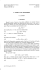### Document 6529975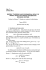### final submission form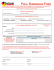### ON INTEGER SEQUENCES### Document 6527798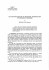### And why this theorem is important for us? Because many... are concerned with finding a maximal (or a minimal) value...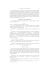### SOME SAMPLE FUNCTION PROPERTIES OF A PROCESS### HOW TO CHARACTERIZE SOME PROPERTIES OF MEASURABLE FUNCTIONS N. Kwami Agbeko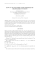### PROBABILITY THEORY - PART 2 INDEPENDENT RANDOM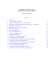### Document 6527827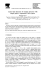### Full text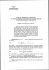### Document 6511833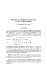### Sample Complexity for Topology Estimation in Networks of LTI Systems ?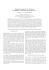### A Uniform Law for Convergence to the Local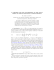### Document 6530657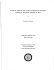### Sample Complexity for Topology Estimation in Networks of LTI Systems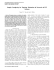### Full text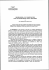### CHAPTER 3: LARGE SAMPLE THEORY CHAPTER 3 LARGE SAMPLE THEORY 1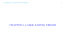### arXiv:1501.02930v1 [math.AP] 13 Jan 2015